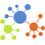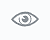# Hierarchical bubbles chartHierarchical bubbles chart

A hierarchical bubbles chart shows hierarchical levels (dimensions) and the proportions (based on a selected measure) through a series of nodes (bubbles) in a network-like structure:

• Hierarchy – Each hierarchy starts from the central root node moving outwards. The first set of nodes are generated based on the first dimension. The child nodes (the second dimension) are placed directly under each parent node of the first set, and so on.

The whole hierarchical group (parent and children) can be differentiated by color (a dimension). For example, the first set of nodes represents years, the second set represents quarters, and the third—months, where all the members belonging to one year have the same color.

• Proportions – The child elements are plotted proportionally based on their measure values. Each parent node represents the total of all its child nodes.

## When to use

Use a hierarchical bubbles chart to show a hierarchical structure of data empathizing how each child node contributes to its parent node and compare it with other nodes within the whole structure. For example, you can evaluate the structure of the company and visualize profit generated by each store and see which products were profitable in each store.

The categories on a chart must be mutually exclusive and not overlapping.

## Data requirements

To build this chart, define the required and optional data fields as follows:

• Dimensions – Up to five dimensions (the structure is built following the order of dimensions)
• Size – (Optional) One measure (to represent the bubble size of each node)
• Tooltip – (Optional) One or more measures
• Color – (Optional) One dimension

## Use case

The following chart shows the countries of each region, where the node size represents the gross sales.Each parent bubble can be collapsible if the respective setting is turned on, on theappearance tab.

Tip: The first dimension is always plotted as the first level of the hierarchy. So, if you want to show a typical hierarchy where the top node is the parent of the lower nodes, you need to add the parent node as the first dimension.

## References

For details on how to customize your visualization, see Visualization settings.

For a whole list of visualizations, see the following topics: